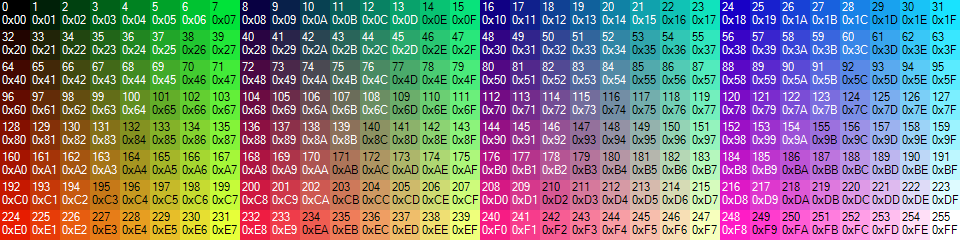DrawShape

This routine is only available on Doors CSE 8.0 and later for the color-screen TI-84 Plus C Silver Edition.

## Technical Details #

### GetPixelA (16-bit, TI-OS VALUES) #

real(7,0,X,Y):
X = x value
Y = y value

This function returns a 16-bit colour code from LCD GRAM in Ans

### GetPixelB (xLIBC palette, TI-OS VALUES) #

real(7,1,X,Y):
X = x value
Y = y value

This function returns a custom xLIB palette colour code from LCD GRAM in Ans

### SetPixelA (16-bit, TI-OS VALUES) #

real(7,2,X,Y,COLOURHIGH,COLOURLOW,SIZE,UPDATELCD):
X = x value
Y = y value
COLOURHIGH = high 8-bits of 16-bit colour code
COLOURLOW = low 8-bits of 16-bit colour code
SIZE = size of pixel to draw
UPDATELCD = 0/1 to update LCD after drawing

This function sets a 16-bit colour pixel in LCD GRAM

### SetPixelB (xLIB palette, TI-OS VALUES) #

real(7,3,X,Y,COLOUR,SIZE,UPDATELCD):
X = x value
Y = y value
COLOUR = colour index (standard xLIB palette)
SIZE = size of pixel to draw
UPDATELCD = 0/1 to update LCD after drawing

This function sets a customer xLIB palette colour pixel in LCD GRAM

### InvertPixel (TI-OS VALUES) #

real(7,4,X,Y,SIZE,UPDATELCD):
X = x value
Y = y value
SIZE = size of pixel to draw
UPDATELCD = 0/1 to update LCD after drawing

This function inverts a pixel in LCD GRAM

### DrawLine (xLIB palette, TI-OS VALUES) #

real(7,5,X0,Y0,X1,Y1,COLOUR,UPDATELCD):
X0 = left x value
Y0 = top y value
X1 = right x value
Y1 = bottom y value
COLOUR = colour index (standard xLIB palette)
UPDATELCD = 0/1 to update LCD after drawing

This function draws a line from X0,Y0 to X1,Y1 using the custom xLIB palette

### InvertLine (TI-OS VALUES) #

real(7,6,X0,Y0,X1,Y1,UPDATELCD):
X0 = left x value
Y0 = top y value
X1 = right x value
Y1 = bottom y value
UPDATELCD = 0/1 to update LCD after drawing

This function draws a line from X0,Y0 to X1,Y1 with inverted colours

### DrawRectangle (xLIB palette, TI-OS VALUES) #

real(7,7,X0,Y0,WIDTH,HEIGHT,COLOUR,UPDATELCD):
X0 = left x value
Y0 = top y value
WIDTH = width of rectangle
HEIGHT = height of rectangle
COLOUR = colour index (standard xLIB palette)
UPDATELCD = 0/1 to update LCD after drawing

This function draws a rectangle starting at X0,Y0 for WIDTH,HEIGHT using the custom xLIB palette

### InvertRectangle (TI-OS VALUES) #

real(7,8,X0,Y0,WIDTH,HEIGHT,UPDATELCD):
X0 = left x value
Y0 = top y value
WIDTH = width of rectangle
HEIGHT = height of rectangle
UPDATELCD = 0/1 to update LCD after drawing

This function draws a rectangle starting at X0,Y0 for WIDTH,HEIGHT with inverted colours

### FillRectangle (xLIB palette, TI-OS VALUES) #

real(7,9,X0,Y0,WIDTH,HEIGHT,COLOUR,UPDATELCD):
X0 = left x value
Y0 = top y value
WIDTH = width of rectangle
HEIGHT = height of rectangle
COLOUR = colour index (standard xLIB palette)
UPDATELCD = 0/1 to update LCD after drawing

This function draws a filled rectangle starting at X0,Y0 for WIDTH,HEIGHT using the custom xLIB palette

### InvertFilledRectangle (TI-OS VALUES) #

real(7,10,X0,Y0,WIDTH,HEIGHT,UPDATELCD):
X0 = left x value
Y0 = top y value
WIDTH = width of rectangle
HEIGHT = height of rectangle
UPDATELCD = 0/1 to update LCD after drawing

This function draws a filled rectangle starting at X0,Y0 for WIDTH,HEIGHT with inverted colours

### DrawCircle (TIOS VALUES) #

XCENTRE = centre x value
YCENTRE = centre y value
COLOUR = colour index (standard xLIB palette)
UPDATELCD = 0/1 to update LCD after drawing

This function will draw a circle (outline only) with its centre at XCENTRE,YCENTRE for RADIUS using the custom xLIB palette

### DrawFilledCircle (TIOS VALUES) #

XCENTRE = centre x value
YCENTRE = centre y value
COLOUR = colour index (standard xLIB palette)
UPDATELCD = 0/1 to update LCD after drawing

This function will draw a filled circled with its centre at XCENTRE,YCENTRE for RADIUS using the custom xLIB palette

### DrawFilledColourRotateRectangle (TIOS VALUES, DCSE8.1) #

real(7,13,X0,Y0,WIDTH,HEIGHT,UPDATELCD
X0 = left x value
Y0 = top y value
WIDTH = width of rectangle
HEIGHT = height of rectangle
UPDATELCD = 0/1 to update LCD after drawing
This function draws a filled rectangle starting at X0,Y0 for WIDTH,HEIGHT. Any pixles that it overlaps will be rotated according to the value of COLOUR_OFFSET (see SETUPCOLORMODE in the SETUP section)\

## Color Values #Special thanks to Shaun ‘Merthsoft’ McFall for generating this image.